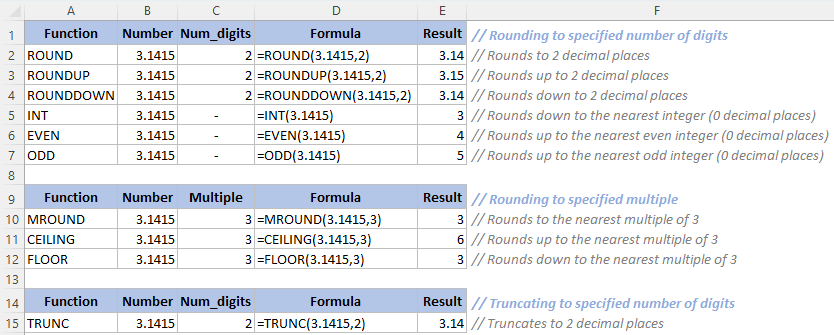## Round up in Excel - A complete beginner's guide

Have you ever needed to round a number up in Excel but weren't sure how to do it? Don't worry, it's a common problem that can be easily solved. In this tutorial, I will show you how to round up numbers to a specific decimal place using Excel's built-in ROUNDUP function as well as a powerful round utility of Kutools. Let's dive in and learn how to round up in Excel!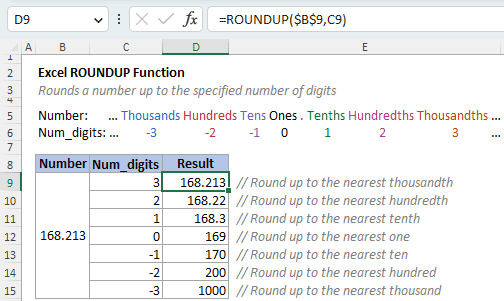#### Round up with ROUNDUP function

The ROUNDUP function always rounds a number up (away from 0) to a specified number of digits. The function is particularly useful when you need to ensure that a result is rounded up to the nearest whole number or a specific decimal place.

##### Syntax of ROUNDUP

=ROUNDUP(number, num_digits)

The ROUNDUP function syntax has the following arguments:

• Number (required): The number to be rounded up.
• Num_digits (required): The number of digits to which you want to round the number up.
• If num_digits is a positive value, ROUNDUP rounds a number up to the right of the decimal point.
 =ROUNDUP(16.143, 2) // Returns 16.15 (16.143 was rounded up to 2 decimal places)
• If num_digits is zero (0), ROUNDUP rounds a number up to the nearest integer.
 =ROUNDUP(16.143, 0) // Returns 17 (16.143 was rounded up to 0 decimal places)
• If num_digits is a negative value, ROUNDUP rounds a number up to the left of the decimal point.
 =ROUNDUP(16.143, -1) // Returns 20 (16.143 was rounded up to 1 decimal place to the left of decimal)
##### Round up to the right of decimal point

To round up values to the right of the decimal point, set num_digits to a positive number. For example, to round the numbers in the following table up to 1, 2, or n decimal places, use 1, 2, or n for the num_digits argument.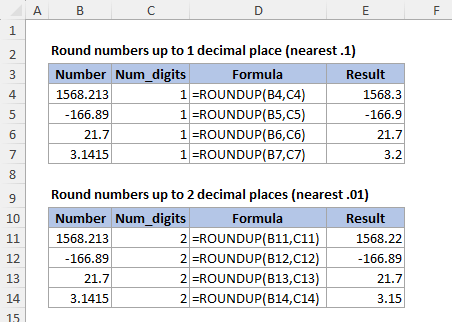##### Round up to an integer

To round up to nearest whole number, set num_digits to 0. For example, to round the numbers in the following table up to the nearest integers, use 0 for the num_digits argument.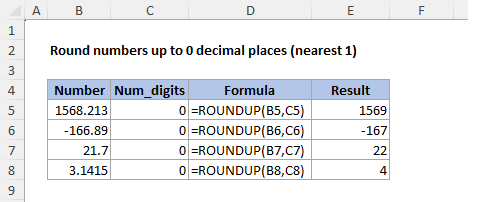##### Round up to the left of decimal point

To round up values to the left of the decimal point, set num_digits to a negative number. For example, to round the numbers in the following table up to 1, 2, or n decimal places to the left of the decimal point, use -1, -2, or -n for the num_digits argument.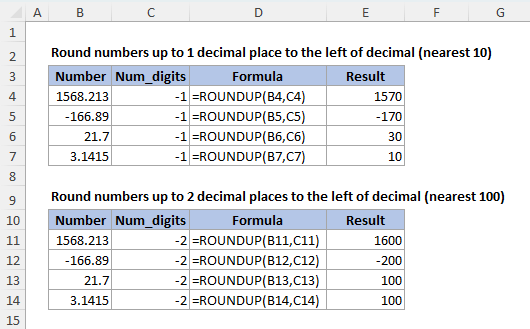Note: ROUNDUP always rounds a number up away from 0, regardless of its sign. When rounding a negative number, its absolute value is first rounded up and then the negative sign (-) is applied back to the rounded result.

##### ROUNDUP Exercises

Deepen your understanding of rounding numbers with ROUNDUP by engaging in the interactive practice exercises provided below. Click on "Show Result " to verify your answers and see the correct results.

Formula Description Result
=ROUNDUP(3.162, 2) Rounds 3.162 up to 2 decimal places (nearest .01) Show Result
=ROUNDUP(53.74, 1) Rounds 53.74 up to 1 decimal place (nearest .1) Show Result
=ROUNDUP(1.68, 0) Rounds 1.68 up to 0 decimal places (nearest 1) Show Result
=ROUNDUP(376.1, 0) Rounds 376.1 up to 0 decimal places (nearest 1) Show Result
=ROUNDUP(436.4, -1) Rounds 436.4 up to 1 decimal place to the left of the decimal point (nearest 10) Show Result
=ROUNDUP(-436.4, -2) Rounds -436.4 up to 2 decimal places to the left of the decimal point (nearest 100) Show Result

#### Batch round up in the original location with Kutools

Kutools for Excel's Round utility offers a simpler solution for rounding up numbers from multiple ranges to a specific decimal place at once, without the need to store the rounded results in another location as required when using the ROUNDUP function. To use this feature, simply select the ranges containing the numbers you wish to round up, and follow the instructions below:

1. On Kutools tab, in Ranges & Cells group, select Round.
2. In the pop-up dialog box, select Rounding up, and then specify the decimal place you want to round the numbers to.
3. Click Ok.
4.5. Note: If you do not have Kutools for Excel installed, please download and install it to enable this Round feature. The professional Excel add-in offers a 30-day free trial with no limitations.

#### Other rounding functions

Rounding to specified number of digits

 ROUND Rounds a number up or down to the specified decimal place (Standard rounding, where numbers less than 5 are rounded down and numbers equal to or greater than 5 are rounded up). ROUNDDOWN Rounds a number down to the specified decimal place. INT Rounds a number down to the nearest integer. EVEN Rounds a number up to the nearest even integer. ODD Rounds a number up to the nearest odd integer.

Rounding to specified multiple

 MROUND Rounds a number up or down to the nearest multiple of a specified significance. CEILING Rounds a number up to the nearest multiple of a specified significance. FLOOR Rounds a number down to the nearest specified multiple.

Truncating to specified number of digits

 TRUNC Truncates a number based on specified number of digits (It actually operates identically to ROUNDDOWN).